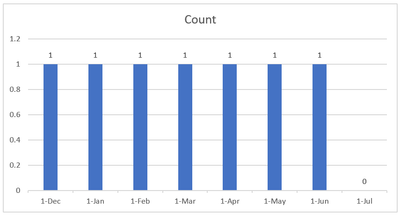cancel
Showing results for
Did you mean:Frequent Visitor

## Count values in consecutive months until a certain date is met

In Power BI desktop, how to count the rows or values from the start date up to the filled date?

For example, my data is like this:I want to create a column chart that would show the count of all open req numbers from Open Date up to the Fill date. So for the table above, the req number should show 1 from 12/1/2021 up to 6/10/2022 and when I select the months beyond the fill date, the number should be 0 since the fill date was met already like the chart below.I don't have any code or dax formula to share right now cause I haven't done anything yet and I honestly don't know how to start.

1 ACCEPTED SOLUTIONCommunity Champion

Hi @nicomathers
You have to create a disconnected date table and measure like :

NEW Recks = calculate(
count(table[req_number]),
Filter (
table,
table[open_Date] <= calculate(max(datetable1[date]) ) &&
table[fill_Date] >= calculate(min(DateTable1[Date]))))
If this post helps, then please consider Accepting it as the solution to help the other members find it more quickly

3 REPLIES 3Community Champion

Hi @nicomathers
You have to create a disconnected date table and measure like :

NEW Recks = calculate(
count(table[req_number]),
Filter (
table,
table[open_Date] <= calculate(max(datetable1[date]) ) &&
table[fill_Date] >= calculate(min(DateTable1[Date]))))
If this post helps, then please consider Accepting it as the solution to help the other members find it more quicklyFrequent Visitor

This is perfect! Thank you so much for your help.Community Champion

I'm happy to help 🙂